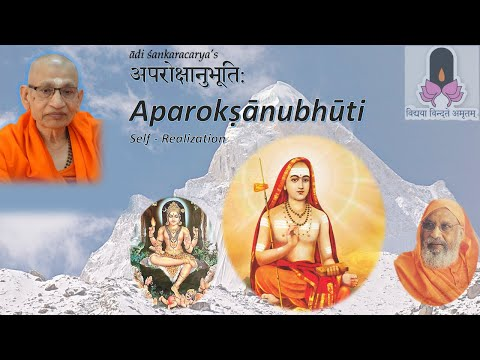Swami Viditatmananda

Swami Viditatmananda

Video/Audio Talks and Lectures

359 classes (~75 mins each)8 classes (search for "Kaivalyopanisad" on the linked page)

Chapter 1
5 classes (~60 mins each)Chapter 2
24 classes (~60 mins each)Chapter 3
7 classes (~60 mins each)Chapter 4
18 classes (~60 mins each)Chapter 5
4 classes (~60 mins each)Chapter 6
20 classes (~60 mins each)Chapter 7
11 classes (~60 mins each)Chapter 8
10 classes (~60 mins each)Chapter 9
7 classes (~60 mins each)Chapter 10
8 classes (~60 mins each)Chapter 11
8 classes (~60 mins each)Chapter 12
8 classes (~60 mins each)Chapter 13
25 classes (~60 mins each)Chapter 14
7 classes (~60 mins each)Chapter 15
7 classes (~60 mins each)Chapter 16
6 classes (~60 mins each)Chapter 17
6 classes (~60 mins each)Chapter 18
32 classes (~60 mins each)Brahma Sutras

First Four Sutras of Brahma Sutras
99 classes (~90 mins each)Explanatory Texts